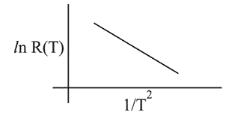# In an experiment, the resistance of a material is plotted as a function of temperature (in some range).Question:

In an experiment, the resistance of a material is plotted as a function of temperature (in some range). As shown in the figure, it is a straight line.One may canclude that:

1. (1) $R(T)=\frac{R_{0}}{T^{2}}$

2. (2) $R(T)=R_{0} \mathrm{e}^{-T_{0}^{2} / T^{2}}$

3. (3) $\mathrm{R}(\mathrm{T})=\mathrm{R}_{0} \mathrm{e}^{-\mathrm{T}^{2} / \mathrm{T}_{0}^{2}}$

4. (4) $\mathrm{R}(\mathrm{T})=\mathrm{R}_{0} \mathrm{e}^{\mathrm{T}^{2} / \mathrm{T}_{0}^{2}}$

Correct Option: 2,

Solution:

(2) Equation of straight line from graph

$\mathrm{y}=-\mathrm{mx}+\mathrm{c}$

$\Rightarrow l \mathrm{nR}=-\mathrm{m}\left(\frac{1}{\mathrm{~T}^{2}}\right)+\mathrm{c}$

here, $m$ & $c$ are constants

$\mathrm{R}=\mathrm{e}^{\left[-\mathrm{m}\left(\frac{1}{\mathrm{~T}^{2}}\right)+\mathrm{c}\right]}$

$=e^{-m\left(\frac{1}{T^{2}}\right)} \times e^{c}$

$R(T)=R_{0} e^{\frac{-T_{0}^{2}}{T^{2}}}$